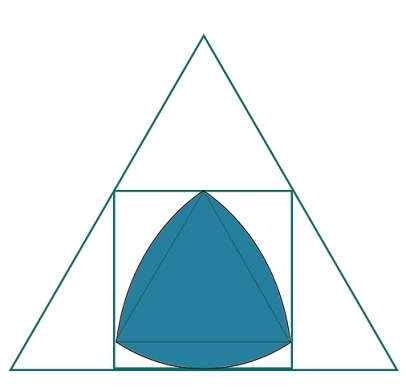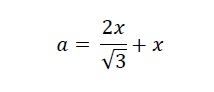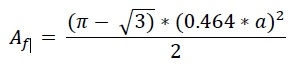# Biggest Reuleaux Triangle inscribed within a Square inscribed in an equilateral triangle?

CServer Side ProgrammingProgramming

Here we will see the area of biggest Reuleax triangle inscribed within a square which is inscribed in an equilateral triangle. Suppose ‘a’ is the side of the triangle. The side of the square is x and the height of the Reuleaux triangle is h.The side of the triangle is −So the value of x is −

𝑥 = 0.464𝑎

The height of the Reuleaux triangle is the same as x. So x = h. So the area of the Reuleaux triangle is −## Example

#include <iostream>
#include <cmath>
using namespace std;
float areaReuleaux(float a) { //side of triangle is a
if (a < 0) //if a is negative it is invalid
return -1;
float area = ((3.1415 - sqrt(3)) * (0.464*a) * (0.464*a))/2;
return area;
}
int main() {
float side = 5;
cout << "Area of Reuleaux Triangle: " << areaReuleaux(side);
}

## Output

Area of Reuleaux Triangle: 3.79311